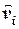USEFUL CONCEPTS GAS LAWS IN THE FREE ATMOSPHERE:   This paper shows how many of the gas laws useful and valid in the laboratory are neither when applied to the free atmosphere.  Many gas laws require that the parcel of air be in a state of equilibrium.  The atmosphere is never really in a state of equilibrium—certainly not when weather is occurring.  Some gas laws require keeping one parameter constant while varying another.  This is not possible in the free atmosphere. THE EQUIPARTITION CONUNDRUM:   The principle of equipartition.   Degrees of freedom.   Allocation of kinetic energies.  Quantum restraints.  Enthalpic heat capacities of air.   The mechanism of equipartition.  How impulse transfer is virtually solely translational.  Transfers of energy. PROBABILITY DENSITY CURVE:   The Probability Density Equation, the mean value, standard deviation, history of the Probability Density Curve, the Normal Curve, velocity distribution along a single Cartesian axis, velocity distribution along the proximity axis, the Error Function Curve, The Complimentary Error Function Curve, proximity velocity curves, Table of Selected Values, evaluating the error functions. MAXWELL’S DEMON EXISTS:  Origin of the term—Maxwell’s letter to Tait.  Essential characteristics of the demon.  The demon in the interface.  Summary. THE SPEED OF SOUND – This paper discusses the Gamma Function and its relation to the speed of sound in gases.  The Gamma Function is shown to be a function of the root-mean-square speed ().  The speed of sound is shown to be the modal impulse velocity (). EQUILIBRIUM AND QUASI-EQUILIBRIUM – This paper points out how statistical mechanics mandates that true equilibrium cannot really exist in real world scenarios.  Three grades of equilibrium are examined:  Molecular Equilibrium, Operational Equilibrium, and Quasi-equilibrium. STILL AIR PARAMETERS – The value of common atmospheric parameters are calculated using a standard of 1,000 hectopascals of atmospheric pressure, a standard temperature of 25°C, and a standard vapor pressure of 10 hectopascals.  Values are then obtained for humid air, dry air, and water vapor.  Parameters evaluated include:  temperature, pressure, number density, mean molecular masses, mean molecular speeds and velocities, mean molecular flows, mean molecular momenta, specific density, mean molecular collisions with surfaces and with one another, mean free paths, mean molecular impulses, and mean kinetic energies of translation. Copyright 2008 by Patrick J. Tyson     www.climates.com  Last revised in August of 2014